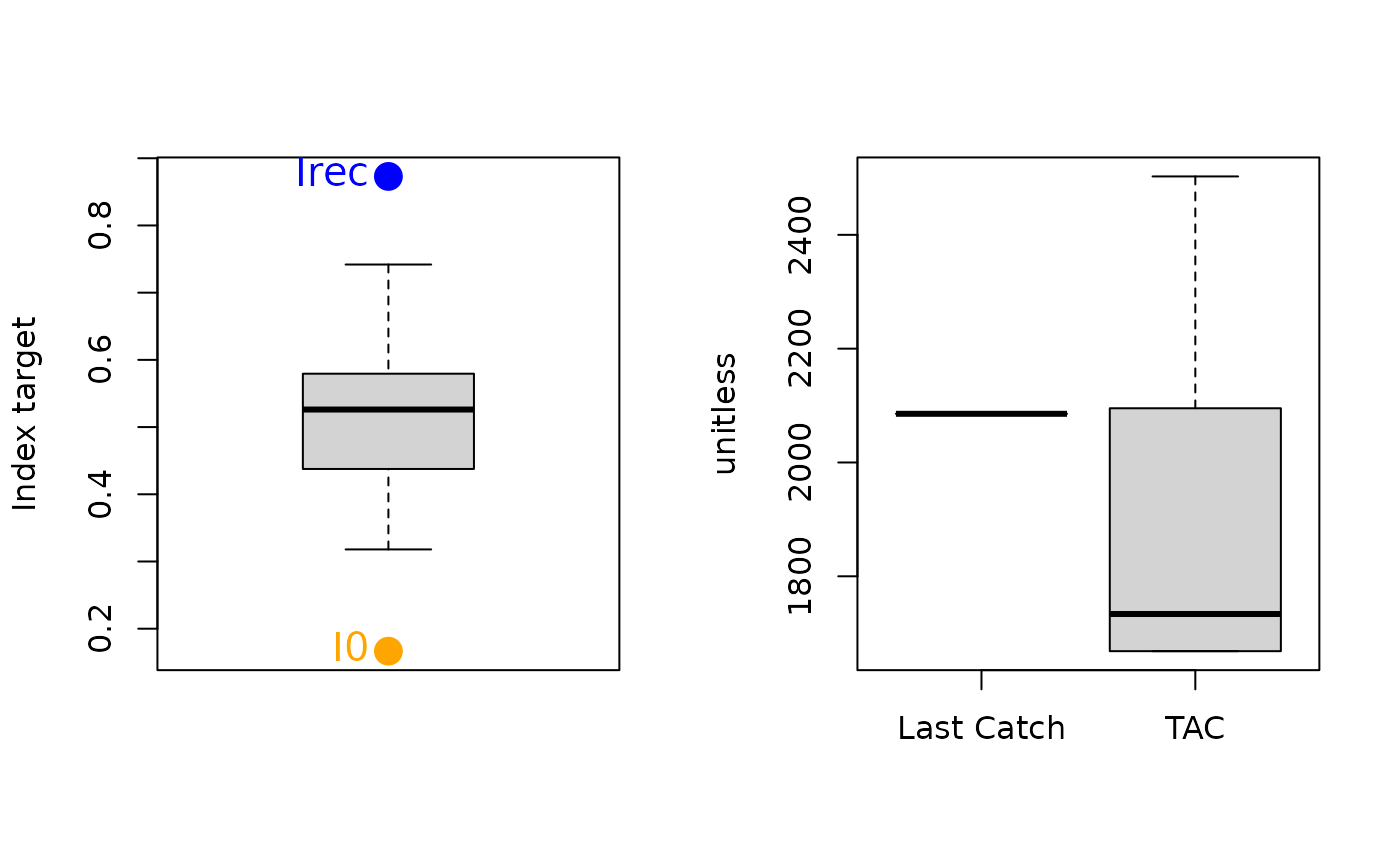An MP similar to SBT2 that modifies a time-series of catch recommendations and aims for target catch rate and catch level based on BMSY/B0 and MSY, respectively.

GB_target(x, Data, reps = 100, plot = FALSE, w = 0.5)

## Arguments

x

A position in the data object

Data

A data object

reps

The number of stochastic samples of the MP recommendation(s)

plot

Logical. Show the plot?

w

A gain parameter

## Value

An object of class Rec-class with the TAC slot populated with a numeric vector of length reps

## Details

The TAC is calculated as: If $$I_\textrm{recent} \geq I_0$$: $$\textrm{TAC}= C_\textrm{ref} \left(w + (1-w)\frac{I_\textrm{rec}-I_0}{I_\textrm{target}-I_0} \right)$$

else: $$\textrm{TAC}= wC_\textrm{ref} \frac{I_\textrm{rec}}{I_0}^2$$

where $$C_\textrm{ref}$$ is a reference catch assumed to be a proxy for MSY (Data@Cref), w is a gain parameter, $$I_\textrm{rec}$$ is the average index over the last 4 years, $$I_\textrm{target}$$ is the target Index (Data@Iref), and $$I_0$$ is 0.2 x the average index over the past 5 years.

In the MSE $$C_\textrm{ref}$$ is the calculated MSY subject to observation error defined in Obs@CV_Cref, and $$I_\textrm{target}$$ is assumed to be the index at MSY subject to observation error (Obs@CV_Iref). Consequently, the performance of this method in the MSE is strongly determined by the specified uncertainty for these parameters.

The TAC is subject to the following conditions:

1. if next TAC > 1.2 last catch, then TAC = 1.2 last catch

2. if next TAC < 0.8 last catch, then TAC = 0.8 last catch

## Required Data

See Data-class for information on the Data object

GB_target: Cref, Ind, Iref

## Rendered Equations

See Online Documentation for correctly rendered equations

Other Index methods: GB_slope(), Gcontrol(), ICI(), Iratio(), Islope1(), Itarget1_MPA(), Itarget1(), ItargetE1()

T. Carruthers

## Examples

 GB_target(1, MSEtool::SimulatedData, plot=TRUE)#> TAC (median)
#>     1705.347